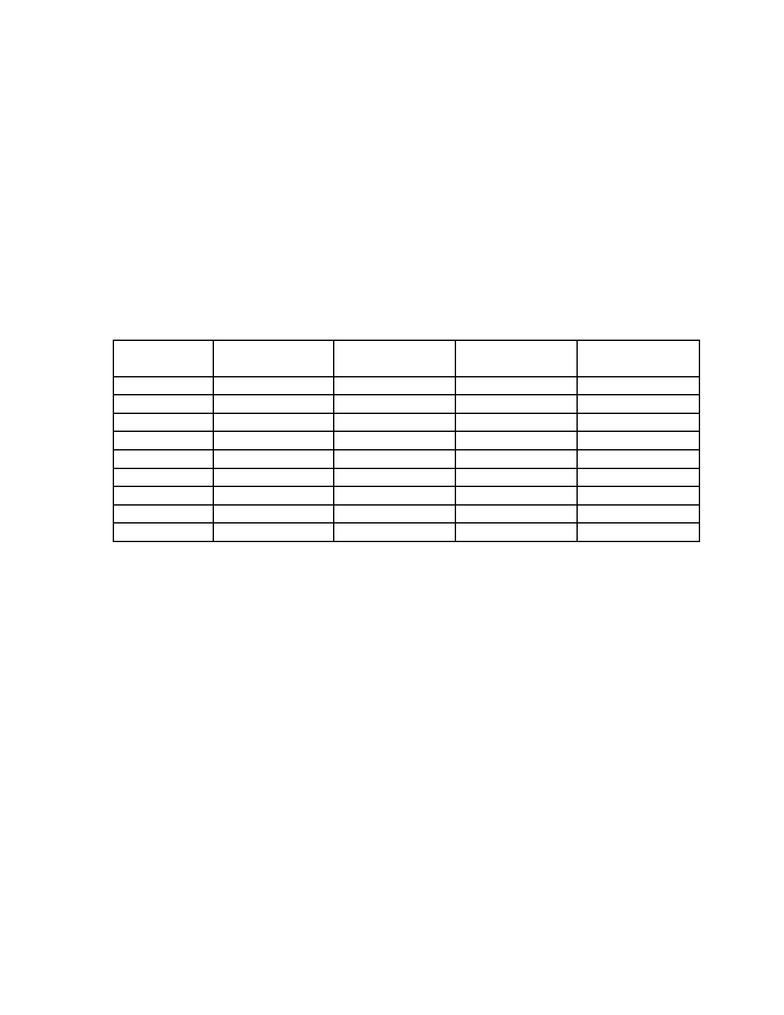Class Notes (1,100,000)
CA (630,000)
UTSG (50,000)
ECO (2,000)
A (10)
Lecture

ECO204Y1 Lecture Notes - Cubic Function, Opportunity Cost, Fixed Capital

Department
Economics
Course Code
ECO204Y1
Professor
A

This preview shows pages 1-3. to view the full 14 pages of the document.Principles of Economics: Short-run Cost
We first examine the cost of firms in the period when capital is fixed to understand the
importance of marginal cost in the determination of profit maximizing output. We do this by
deriving cost functions from production functions.
SHORT-RUN PRODUCTION AND COST FUNCTIONS
Production Function
Definition: The set of all maximum possible outputs from a given input or inputs that provide a
technologically feasible way to produce a commodity or service.
Q = f(Xo, X1, X2, X3, X4, … XN) where Xi represents the inputs into production
We simplify this by dividing the inputs into Capital (K) and Labour (L) to get
Q = f(L, K)
Firm
Definition: A business organization producing goods and services from resource inputs
‘Business’ implies the assumption of profit maximization
The firm connects costs and output as the smallest unit of production.
Industry
Definition: All the firms that produce a given good or service
Short-run
Definition: The period in which one factor (input) is fixed
Long-run
Definition: The period in which all factors are variable
Variable Inputs
Definition: Inputs that vary in quantity with each additional unit of output
- 1 -

Only pages 1-3 are available for preview. Some parts have been intentionally blurred.Principles of Economics: Short-run Cost
Variable inputs include Labour, Fuel, and Raw Materials but we use Labour (L) to represent
them all
Fixed Inputs
Definition: Inputs that do not vary in quantity with an additional unit of output
Fixed inputs include equipment and machinery, buildings, and land but we use Capital (K) to
represent them all
SHORT-RUN PRODUCTION FUNCTIONS
Total Product of Labour (TPL)
Definition: The total output produced from each total labour output given fixed capital
TPL = q = f(LK)
(Q = output of the industry and q = output of a firm so that Q = nq where n = #firms in the
industry)
Average Product of Labour (APL or simply AP since we will assume that capital is fixed)
Definition: Average output of each labour unit given fixed capital
APL = q/L
Marginal Product of Labour (MPL or simply MP)
Definition: The change in output due to a change in labour given fixed capital
MPL = q/L [(q1 – q0)/(L1 – L0) for discrete changes]
(or = dq/dL for infinitesimal changes in Labour)
NOTE: The Marginal Product of Labour is often expressed as the change in output due to an
additional ‘unit’ of labour.
- 2 -

Only pages 1-3 are available for preview. Some parts have been intentionally blurred.Principles of Economics: Short-run Cost
Law of Eventually Diminishing Returns
Definition: Marginal Product eventually decreases with increases in labour since the efficiency of
additional labour eventually falls due to fixed capital
e.g. The following table shows the Total Product, Average Product, and Marginal Products for
units (days) of labour at cotton cloth manufacturer with a spinning, weaving, and dyeing
machine.
The equation q = - L3 + 12L2 describes the total product of labour.
Labour Units Total Product
(TP)
Average Product
(AP)
Marginal Product
MP = Ln+1 - Ln
Marginal Product
MP = dTP/dL
1 11 11 11 21
2 40 20 29 36
3 81 27 41 45
4 128 32 47 48
5 175 35 47 45
6 216 36 41 36
7 245 35 29 21
8 256 32 11 0
9 243 27 -13 -27
Marginal Product initially rises because additional workers can use the fixed capital more
efficiently but eventually marginal product declines because the fixed capital limits the
productivity of the workers. Marginal Product declines to below 0 when an additional worker
results in a fall in Total Product, not just a decline in Marginal Product.
Notice that Total Product shows us that output increases up to 8 workers and then decreases
but does not show us the rate at which Total Product increases or decreases. Marginal Product is
more informative since positive (negative) Marginal product tells us not only whether Total
Product increases (decreases) but also the change in Total Product.
The diagram below shows the output as a function of Labour in the upper diagram and output
per worker as a function of Labour in the lower diagram. Total product is a function of total labour
- 3 -
You're Reading a Preview

Unlock to view full version